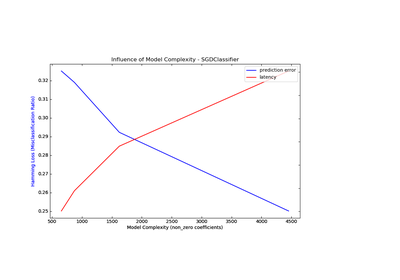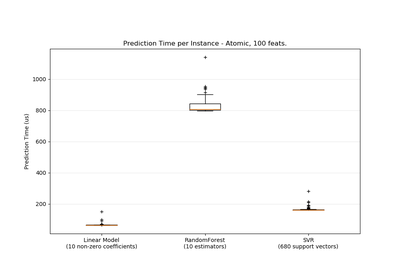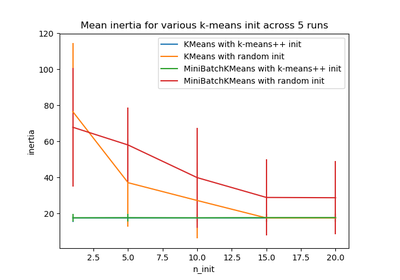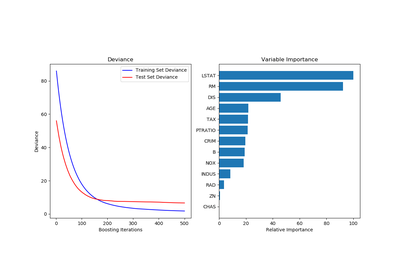# `sklearn.utils`.shuffle¶

`sklearn.utils.``shuffle`(*arrays, **options)[source]

Shuffle arrays or sparse matrices in a consistent way

This is a convenience alias to `resample(*arrays, replace=False)` to do random permutations of the collections.

Parameters: *arrays : sequence of indexable data-structures Indexable data-structures can be arrays, lists, dataframes or scipy sparse matrices with consistent first dimension. random_state : int, RandomState instance or None, optional (default=None) The seed of the pseudo random number generator to use when shuffling the data. If int, random_state is the seed used by the random number generator; If RandomState instance, random_state is the random number generator; If None, the random number generator is the RandomState instance used by np.random. n_samples : int, None by default Number of samples to generate. If left to None this is automatically set to the first dimension of the arrays. shuffled_arrays : sequence of indexable data-structures Sequence of shuffled views of the collections. The original arrays are not impacted.

Examples

It is possible to mix sparse and dense arrays in the same run:

```>>> X = np.array([[1., 0.], [2., 1.], [0., 0.]])
>>> y = np.array([0, 1, 2])

>>> from scipy.sparse import coo_matrix
>>> X_sparse = coo_matrix(X)

>>> from sklearn.utils import shuffle
>>> X, X_sparse, y = shuffle(X, X_sparse, y, random_state=0)
>>> X
array([[ 0.,  0.],
[ 2.,  1.],
[ 1.,  0.]])

>>> X_sparse
<3x2 sparse matrix of type '<... 'numpy.float64'>'
with 3 stored elements in Compressed Sparse Row format>

>>> X_sparse.toarray()
array([[ 0.,  0.],
[ 2.,  1.],
[ 1.,  0.]])

>>> y
array([2, 1, 0])

>>> shuffle(y, n_samples=2, random_state=0)
array([0, 1])
```

## Examples using `sklearn.utils.shuffle`¶Model Complexity InfluencePrediction LatencyColor Quantization using K-MeansEmpirical evaluation of the impact of k-means initialization